# Engineering

Hidromec, grazie alle competenze del suo staff è in grado di ideare, progettare e costruire, manutenere ed implementare cilindri per le vostre specifiche applicazioni.
Per Engineering intendiamo una precisa metodologia di realizzazione e di controllo di conformità: un risultato difficile da ottenere. Un modello collaudato e certificato ISO9001 si sviluppa attraverso diverse fasi: va dalla verifica e controllo dei materiali di ogni singolo componente, all’analisi dei rivestimenti superficiali, fino a giungere al Reverse Engineering dei sottocomponenti, il tutto per ottenere la massima qualità e conformità del prodotto e dei suoi sottocomponenti, e creare un database di conformità.

Il cindro Idraulico (conosciuto anche con il nome di motore idraulico lineare) è un dispositivo di attuazione meccanico utilizzato per imprimere un movimento unidirezionale tramite un dispositivo a corsa lineare. Ha molteplici applicazioni, sopratturo nella costruzione di apparecchiature meccaniche e nella progettazione di veicoli, macchinari industriali e applicazioni di ingegneria civile.

#### Buckling

Special problems to do with stability occur when cylinders with long stroke lengths are used. For the purpose of calculation these cases are divided into areas:

• Non elastic buckling loads (Tetmajer’s calculation) and n
• Elastic or Hook’s buckling loads (its critical limiting load is determined
by Euler’s equation) n

In hydraulic cylinder Euler’s calculation is basically the calculation used, as the piston rod may
usually be considered to be a slender strut (negligible diameter).

• Buckling load K= ………In N (1) i.e. the rod buckles under this load
• Max. operating load F = K/S In N (2)
• Sk = free buckling length in mm
• E = modulus of elasticity (2.1 x 10 * for steel ) in N/mm
• J = moment of inertia for circular cross-section in mm* …..
• S = safety factor (3.5)

The length to be used as the free buckling length may be determined from the Euler loading cases (see table 4). In order to simplify the calculation the stiffening due to the
cylinder tube is ignored. This provides the required safety margin in standard cylinders, the installation position of which is usually not known, in order to cater for any superimposed bending loads.

 Euler’s loading case Case 1 One end free, one end rigidly connected. Case 2 (Basis case) Two ends pivoted. Case 3 One end pivoted, one end rigidly connected. Case 4 Two ends rigidly connected. Illustration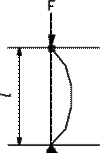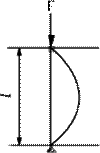Free buckling length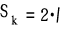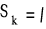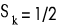Installation position for cylinder NoteMounting type FA,FB,LA,LB, MF3, ME7,MF4,ME8,MS2Mounting type TA,TC,CA,CB,MP3,MP1, MT4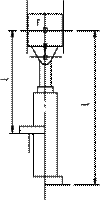Mounting typeFA,FB,LA,LB,MF3,ME7, MF4,ME8,MS2 Load must be carefully guided, or else possible bracing.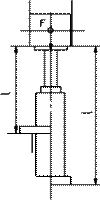Mounting type FA,FB,LA,LB,MF3,ME7, MF4,ME8,MS2 Not suitable, as  bracing is to be expected.

## Hydraulic force - area formulas and calculatorThe force produced by a double acting hydraulic piston on the rod side can be expressed as

F1 = (π (d22 - d12) / 4) P1         (1)

where

F1 = rod pull force (lb, N)

d1 = rod diameter (in, m)

d2 = piston diameter (in, m)

P1 = pressure in the cylinder (rod side) (lff/in2 (psi), N/m2 (Pa))

The force produced opposite the rod can be expressed as

F2 = (π d22 / 4) P2         (2)

where

F2 = rod push force (lb, N)

P2 = pressure in the cylinder (opposite rod) (lff/in2 (psi), N/m2 (Pa))

### Push Diagram

Rod pushing force for hydraulic cylinders are indicated below:• 1 psi (lb/in2) = 144 psf (lbf/ft2) = 6,894.8 Pa (N/m2) = 6.895x10-3 N/mm2 = 6.895x10-2 bar
• 1 N/m2 = 1 Pa = 1.4504x10-4 lb/in2 = 1x10-5 bar = 4.03x10-3 in water = 0.336x10-3 ft water = 0.1024 mm water = 0.295x10-3 in mercury = 7.55x10-3 mm mercury = 0.1024 kg/m2 = 0.993x10-5 atm
• 1 lbf (Pound force) = 4.44822 N = 0.4536 kp
• 1 N (Newton) = 0.1020 kp = 7.233 pdl = 7.233/32.174 lbf = 0.2248 lbf = 1 (kg m)/s2 = 105 dyne = 1/9.80665 kgf
• 1 in (inch) = 25.4 mm
• 1 m (meter) = 39.37 in = 100 cm = 1000 mm

### Pull Diagram

Rod pulling force for hydraulic cylinders are indicated below:#### Rod

Piston Rod Size Selection

The selection of a piston rod for thrust (push) conditions requires the following steps to be carried out:

1. Determine the type of cylinder mounting style and rod end connection to be used. Consult the Stroke Factor table and determine which factor corresponds to the application.
2. Using this stroke factor, determine basic length from the equation:
Basic Length = Net Stroke x Stroke Factor
( The Piston Rod Selection Chart, below, is prepared for standard rod extensions beyond the face of the gland retainer. For rod extensions greater than standard’ add the increase to the stroke to arrive at the basic length’)
3. Find the load imposed for the thrust application by multiplying the full bore area fo the cylinder by the system pressure, or by referring to the Push and Pull Force charts.
4. Using the Piston Rod Selection Chart below, look along the values of ‘ basic length ’ and ‘ thrust ‘ as found in 2. and 3. above, and note the point of intersection.

The correct piston rod size is read from the diagonally curved line labeled ‘Rod Diameter‘ above the point of intersection.Long Stroke and Stop Tubes

When considering the use of long stroke cylinders, the piston rod should be of sufficient diameter to provide the necessary column strength.
For tensile(pull)loads, the rod size is selected by specifying standard cylinders with standard rod diameters and using them at or below the rated pressure.

For long stroke cylinders under compressive loads, the use of stop tubes should be considered, to reduce bearing stress. Selection of a stop tube is described.#### Size

CALCULATION OF CYLINDER DIAMETER

Given that the load and operating pressure of the system are known, and that a piston rod size has been estimated taking account of whether the rod is in tension(pull) or compression (push), then the cylinder bore can be selected.
If the piston rod is in compression, use the ‘Push Force’ table below.

1. Identify the operating pressure closest to that required;
2.  In the same column, identify the force required to move the load (always rounding up);
3.  In the same row, lock along to the cylinder bore required.

If the cylinder envelope dimensions are too large for your application increase the operating pressure, if possible, and repeat the exercise.

Push Force

 Bore φ ㎜ Cylinder Bore Area ㎟ Cylinder Push Force in kN 10 Bar 40 Bar 63 Bar 100 Bar 125 Bar 160 Bar 40 1257 1.3 5.0 7.9 12.6 15.7 20.1 50 1964 2.0 7.9 12.4 19.6 24.6 31.4 63 3118 3.1 12.5 19.6 31.2 39.0 49.9 80 5027 5.0 20.1 31.7 50.3 62.8 80.4 100 7855 7.9 31.4 49.5 78.6 98.2 126 125 12272 12.3 49.1 77.3 123 15.3 196 160 20106 20.1 80.4 127 201 251 322 200 31426 31.4 126 198 314 393 503 250 49087 49.1 196 309 491 614 785 320 80425 80.4 322 507 804 1005 1287

If the piston rod is in tension, use the ‘Deduction for Pull Force’ table. The procedure is the same but, due to the reduced piston surface area resulting from the piston rod, the force available on the ‘pull’ stroke will be smaller, To determine the pull force.

1. Follow the procedure given for ‘push’ applications as described above.
2.  Using the ‘Deduction for Pull Force’ table below, establish the force indicated according to the rod diameter and pressure selected.
3. Deduct this from the original ‘Push’ force. The resultant is the net force available to move the load.

If this force is not large enough, go through the process again but increase the system operating pressure or cylinder diameter if possible. If in doubt, Our design engineers will be pleased to assist.

Deduction for Pull Force

 Piston Rod φ ㎜ Piston Rod Area ㎟ Reduction in Force 10 Bar 40 Bar 63 Bar 100 Bar 125 Bar 160 Bar 22 380 0.4 1.5 2.4 3.8 4.8 6.1 28 616 0.6 2.5 3.9 6.2 7.7 9.9 36 1018 1.0 4.1 6.4 10.2 12.7 16.3 45 1590 1.6 6.4 10.0 15.9 19.9 25.5 56 2463 2.5 9.9 15.6 24.6 30.8 39.4 70 3848 3.8 15.4 24.2 38.5 48.1 61.6 90 6362 6.4 25.5 40.1 63.6 79.6 102 110 9503 9.5 38.0 59.9 95.1 119 152 140 15394 15.4 61.6 97.0 154 193 246 180 25447 25.4 102 160 254 318 407 220 38013 38.0 152 240 380 475 608